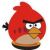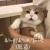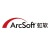0+0+0+0+0+0+0+0+0+0+0+0+0+0+0+0+0+0+0+0+0+0+0+0+0+0+0+0+0+0+0+0+0+Machine learning-based lithography hotspot detection has been deeply studied recently, from varies feature extraction techniques to efficient learning models. It has been observed that such machine learning-based frameworks are providing satisfactory metal layer hotspot prediction results on known public metal layer benchmarks. In this work, we seek to evaluate how these machine learning-based hotspot detectors generalize to complicated patterns. We first introduce a automatic layout generation tool that can synthesize varies layout patterns given a set of design rules. The tool currently supports both metal layer and via layer generation. As a case study, we conduct hotspot detection on the generated via layer layouts with representative machine learning-based hotspot detectors, which shows that continuous study on model robustness and generality is necessary to prototype and integrate the learning engines in DFM flows. The source code of the layout generation tool will be available at https://github. com/phdyang007/layout-generation.0+0+0+下载预览0+0+0+0+0+0+The objective of this work is to improve the accuracy of building demand forecasting. This is a more challenging task than grid level forecasting. For the said purpose, we develop a new technique called recurrent transform learning (RTL). Two versions are proposed. The first one (RTL) is unsupervised; this is used as a feature extraction tool that is further fed into a regression model. The second formulation embeds regression into the RTL framework leading to regressing recurrent transform learning (R2TL). Forecasting experiments have been carried out on three popular publicly available datasets. Both of our proposed techniques yield results superior to the state-of-the-art like long short term memory network, echo state network and sparse coding regression.0+0+0+下载预览
Top